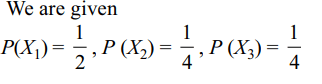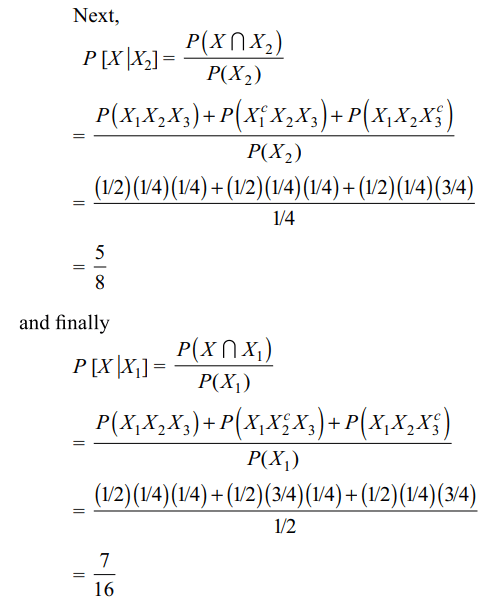## Probability Questions and Answers Part-6

1. For two events A and B, if P(A) = P(A | B) = 1/4 and P(B | A) = 1/2, then
a) A and B are independent
b) P (A' | B) = 3/4
c) P (B' | A') = 1/2.
d) All of the Above

Explanation:2. If A and B are two independent events such that $P\left(A' \cap B\right)=2/15$     and $P\left(A \cap B'\right)=1/6$   , then P(B) is
a) 1/5
b) 1/6
c) 4/5
d) Both b and c

Explanation: Since A and B are independent3. A student appears for tests I, II, and III. The student is successful if he passes either in tests I and II or tests I and III. The probabilities of the student passing in tests I, II, III are p, q and 1 2, respectively. If the probability that the student is successful is 1/2, then
a) p = 1, q = 0
b) p = 2/3, q = 1/2
c) p = 3/5, q = 2/3
d) All of the Above

Explanation: Let A, B and C be the events that the student is successful in tests I, II and III, respectively. Then
P(the student is successful)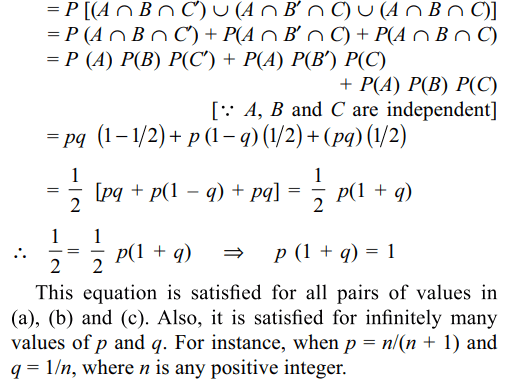4. Let X be a set containing n elements. If two subsets A and B of X are picked at random, the probability that A and B have the same number of elements is
a) $\frac{^{2n}C_{n}}{2^{2n}}$
b) $\frac{1}{^{2n}C_{n}}$
c) $\frac{1.3.5...\left(2n-1\right)}{2^{n}.\left(n !\right)}$
d) Both a and c

Explanation: We know that the number of subsets of a set5. Let E and F be two independent events. The probability that both E and F happen is 1/12 and the probability that neither E nor F happens is 1/2. Then
a) P(E) = 1/3, P(F) = 1/4
b) P(E) = 1/2, P(F) = 1/6
c) P(E) = 1/4, P(F) = 1/3
d) Both a and c

Explanation: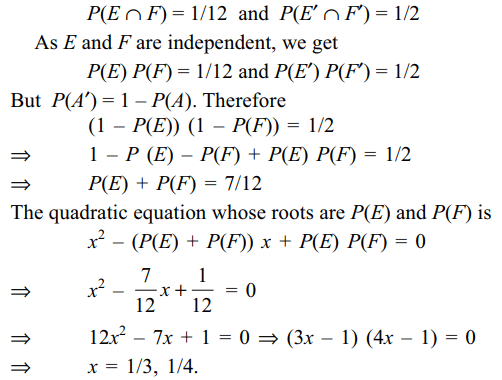6. A random variable X follows binomial distribution with mean a and variance b. Then
a) a > b > 0
b) $\frac{a}{b}>1$
c) $\frac{a^{2}}{a-b}$   is an integer
d) All of the Above

Explanation: Suppose X ~ B(n, p). Then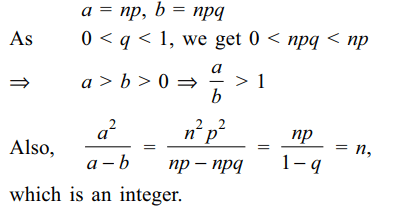7. If A1,A2,....,An are n independent events such that $P\left(A_{i}\right)=\frac{1}{i+1},i=1,2,....,n$      . The probability that none of A1,A2,....,An occurs is
a) $\frac{n}{n+1}$
b) $\frac{1}{n+1}$
c) less than $\frac{1}{n}$
d) Both b and c

Explanation: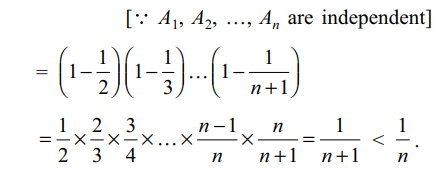8. A four digit number (numbered from 0000to 9999) is said to be lucky if the sum of first two digits is equal to the sum of its last two digits. If a four digit number is picked up at random, then the probability that it is lucky is
a) > 0.065
b) < 0.068
c) 0.067
d) All of the Above

Explanation: The total number of ways of choosing the ticket is 1000.9. A bag contains n $\left(\geq 7\right)$  balls number 1, 2, … n. Four balls are taken out at random without replacement. Let pn denote the probability that all the four balls bear odd numbers, then
a) $P_{n}=\frac{\left(n+1\right)\left(n-5\right)}{16n\left(n-3\right)}$       if n is odd
b) $P_{n}=\frac{\left(n-4\right)\left(n-6\right)}{16n\left(n-1\right)\left(n-3\right)}a$       if n is even
c) $P_{20}<\frac{7}{23}$
d) All of the Above

Explanation: Let n = 2k + 1, k ≥ 310. A ship is fitted with three engines $E_{1},E_{2}$ and $E_{3}$. The engines function independently of each other with respective probabilities $\frac{1}{2},\frac{1}{4}$   and $\frac{1}{4}$ For the ship to be operational at least two of its engines must function. Let X denote the event that the ship is operational and let $X_{1},X_{2}$ and $X_{3}$ denote respectively the events that the engines $E_{1},E_{2}$ and $E_{3}$ are functioning. Which of the following is (are) true?
a) $P\left[X_1^c \mid X\right]=\frac{3}{16}$
b) P [Exactly two engines of the ship are functioning | X] = $\frac{7}{8}$
c) $P\left[X \mid X_{1}\right]=\frac{7}{16}$
d) Both b and c